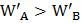# Two springsandare identical butis harder than. Letandrepresent the work done when the springs are stretched through the same distance andandare the work done when these are stretched by equal forces, then which of the following is true a)andb)andc)andd)and## Question ID - 50855 :- Two springsandare identical butis harder than. Letandrepresent the work done when the springs are stretched through the same distance andandare the work done when these are stretched by equal forces, then which of the following is true a)andb)andc)andd)and3537

 (b)is the sameForces are the sameAsand;butNext Question :

There were 24 students in a class. One of them, who was 18 years old, left the class and his place was filled up by a newcomer. If the average of the class thereby, was lowered by one month, the age of the newcomer is

(a)  14 years             (b)15 years               (c)16 years               (d) 17 years